KFUPM-Physics102-052
Dr.Aljalal
Sec16
Sec17
Sec18

Recitation ch 27

Question 1
The resistance between points a and b in figure 5 is
60 ohms. What is the value of R ?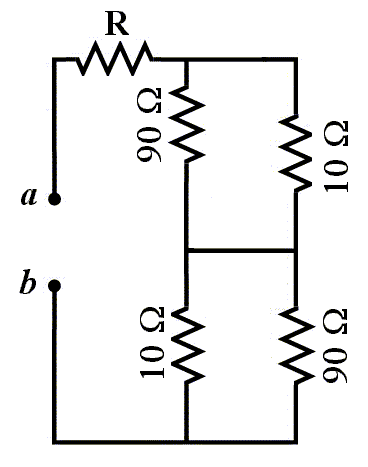a   50 ohms
b   10 ohms
c   42 ohms
d   18 ohms
e   0.5 ohms
==============================================
Question 2
In figure (5), all the resistors have a value of 2 Ohms. The
battery is ideal with an emf = 15 V. What is the potential
difference across the resistor R3?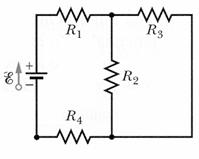a   1.5 Volts.
b   5.0 Volts.
c   15 Volts.
d   3.0 Volts.
e   2.5 Volts.
==============================================
Question 3
In figure 6: R1 = 2.0 ohms, R2 = 4.0 ohms, R3 = 8.0 ohms,
E1 = 2.0 volts, E2 = 4.0 volts and E3 = 6.0 volts.
What is the rate of energy dissipation in R1 ?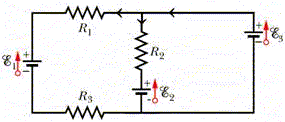a   0.16 W
b   0.32 W
c   0.24 W
d   0.08 W
e   0.40 W
==============================================
Question 4
What is the power dissipated in 4.0 Ohm resistor in Figure 4.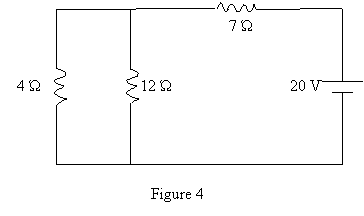a   9.0 W.
b   4.3 W.
c   1.2 W.
d   3.0 W.
e   6.0 W.
==============================================
Question 5
A battery is connected across a series combination
of two identical resistors. If the potential difference
across the terminals of the battery is V, and the current
in the battery is I, then:

a   the potential difference across each resistor is V
and the current in each resistor is I/2.
b   the potential difference across each resistor is V/2
and the current in each resistor is 2I.
c   the potential difference across each resistor is V
and the current in each resistor is I.
d   the potential difference across each resistor is V/2
and the current in each resistor is I/2.
e   the potential difference across each resistor is V/2
and the current in each resistor is I.
==============================================
Question 6
In figure (8), what is the potential difference Va-Vb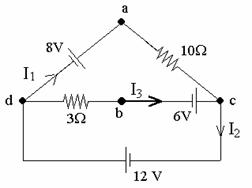a   8 V.
b   10 V.
c   26 V.
d   6 V.
e   2 V.
==============================================
Question 7
In the circuit shown in figure 3, the capacitor was
initially uncharged. At time t = 0, switch S is closed.
If T denotes the time constant, the current through the
3-ohm resistor at t = T/10 is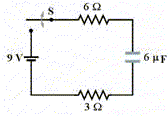a   0.90 A.
b   1.5 A.
c   2.5 A.
d   zero.
e   3.0 A.
==============================================
Question 8
At t=0, a 2.0*10**(-6) Farad capacitor is connected in series
to a 20-V battery and a 2.0*10**6 Ohm resistor. How long does
it take for the potential difference across the capacitor to
be 12 V?

a   3.7 s.
b   2.8 s.
c   1.2 s.
d   0.6 s.
e   2.0 s.
==============================================
Question 9
In figure 5, the current in the 5.0-ohm resistor is 3.0 A.
What is the potential difference Va - Vb ?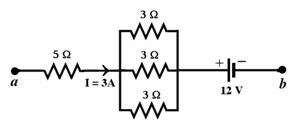a   + 30 V
b   + 54 V
c   - 30 V
d   + 6.0 V
e   - 6.0 V
==============================================
Question 10
A portion of a circuit is shown in figure (6), with the
values of the currents given for some branches. What is
the direction and value of the current I?a   Down, 2 A.
b   Down, 4 A.
c   Up, 6 A.
d   Down, 6 A.
e   Up, 4 A.
==============================================
Question 11
Calculate the power dissipated in the 6.0-ohm
resistor in figure 6.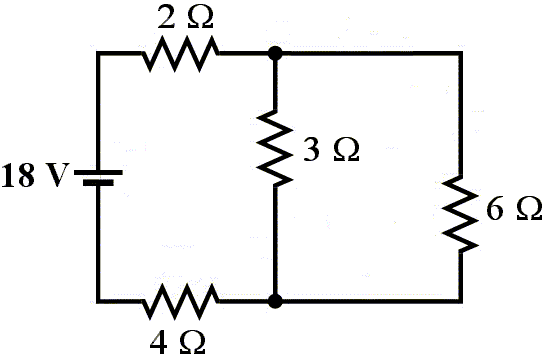a   1.5 W
b   1.7 W
c   2.3 W
d   3.4 W
e   30 W
==============================================
Question 12
The current in the 5.0-ohm resistor in the circuit shown
in figure (7) is: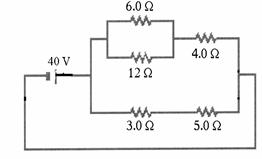a   3.0 A.
b   0.67 A.
c   5.0 A.
d   2.4 A.
e   0.42 A.
==============================================
Question 13
For the circuit shown in figure 4, find the potential
difference VA - VB, if I1 = 3.0 A and I3 = 4.0 A.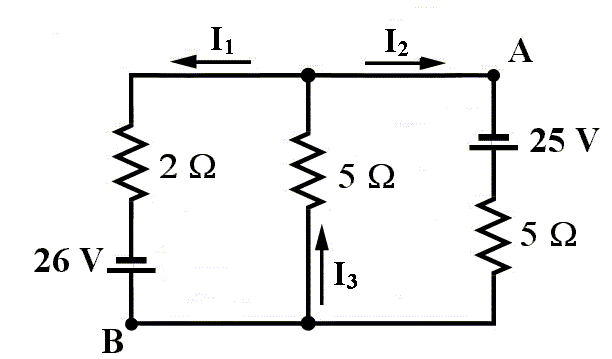a   - 30 V
b   + 32 V
c   + 20 V
d   - 32 V
e   - 20 V
==============================================
Question 14
In figure 3, if R = 10 Ohm find the current in R.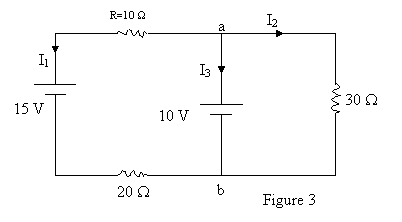a   - 0.2 A.
b   - 1.1 A.
c   0.4 A.
d   0.2 A.
e   - 0.4 A.
==============================================
Question 15
A certain capacitor, in series with a 720-ohm resistor, is
being charged. At the end of 10 milliseconds, its charge
is 50 % of the maximum charge.
The capacitance is:

==============================================
Question 16
In figure 2, a battery of emf of 12-Volt and internal
resistance of r = 3.0 Ohm is connected to a bulb of
resistance R. If the bulb will light at a steady current
of 0.1 A, what should the value of R be?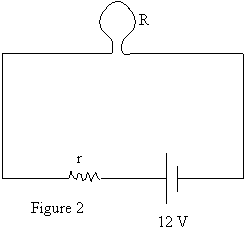a   200 Ohm.
b   130 Ohm.
c   40 Ohm.
d   117 Ohm.
e   35 Ohm.
==============================================
Question 17
A resistor R = 30*10**6 Ohm is connected in series with
a capacitor C = 3.0 micro-F and a 21-Volt battery for
long time. The battery was removed, then R and C are
connected in a loop. What is the energy stored in the
capacitor C after one minute?

a   204 micro-J.
b   24 micro-J.
c   47 micro-J.
d   174 micro-J.
e   11 micro-J.
==============================================

1     c
2     d
3     b
4     a
5     e
6     c
7     a
8     a
9     a
10     d
11     d
12     c
13     e
14     a
15     a
16     d
17     d